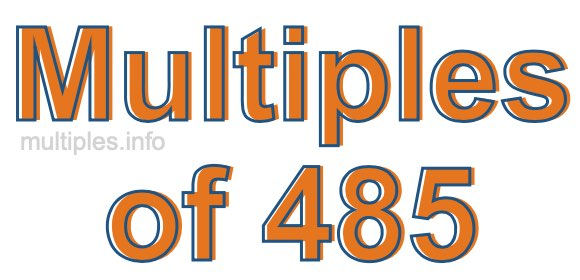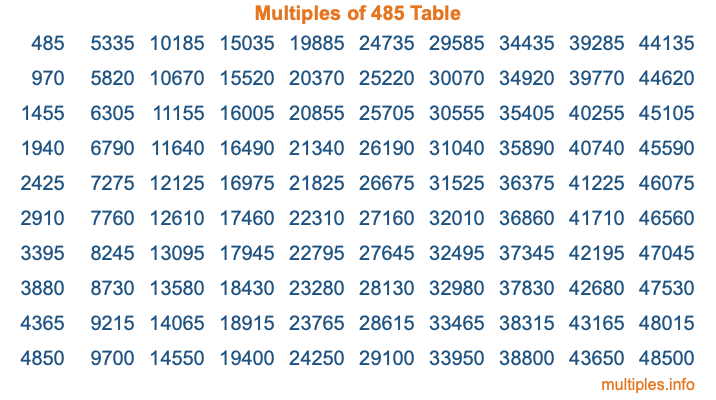Multiples of 485Welcome to the Multiples of 485 page. Here we will first teach you everything you will ever need to know about the multiples of 485, and then give you a study guide summary of everything we taught you to make sure you remember it all. Use this page to look up facts and learn information about the multiples of 485. This page will make you a multiples of four hundred eighty-five expert!

Definition of Multiples of 485
Multiples of 485 are all the numbers that when divided by 485 equal an integer. Each of the multiples of 485 are called a multiple. A multiple of 485 is created by multiplying 485 by an integer.

Therefore, to create a list of multiples of 485, you start with 1 multiplied by 485, then 2 multiplied by 485, then 3 multiplied by 485, and so on for as long as you want. Thus, the list of the first five multiples of 485 is 485, 970, 1455, 1940, and 2425. To see a larger list of multiples of 485, see the printable image of Multiples of 485 further down on this page. We also have a category where you can choose any nth multiple of 485.

Multiples of 485 Checker
The Multiples of 485 Checker below checks to see if any number of your choice is a multiple of 485. In other words, it checks to see if there is any number (integer) that when multiplied by 485 will equal your number. To do that, we divide your number by 485. If the the quotient is an integer, then your number is a multiple of 485.

Is  a multiple of 485?

Least Common Multiple of 485 and ...
A Least Common Multiple (LCM) is the lowest multiple that two or more numbers have in common. This is also called the smallest common multiple or lowest common multiple and is useful to know when you are adding our subtracting fractions. Enter one or more numbers below (485 is already entered) to find the LCM.

Check out our LCM Calculator if you need more details about the Least Common Multiple or if you need the LCM for different numbers for adding and subtraction fractions.

nth Multiple of 485
As we stated above, 485 is the first multiple of 485, 970 is the second multiple of 485, 1455 is the third multiple of 485, and so on. Enter a number below to find the nth multiple of 485.

th multiple of 485

Multiples of 485 vs Factors of 485
485 is a multiple of 485 and a factor of 485, but that is where the similarities end. All postive multiples of 485 are 485 or greater than 485. All positive factors of 485 are 485 or less than 485.

Below is the beginning list of multiples of 485 and the factors of 485 so you can compare:

Multiples of 485: 485, 970, 1455, 1940, 2425, etc.

Factors of 485: 1, 5, 97, 485

As you can see, the multiples of 485 are all the numbers that you can divide by 485 to get a whole number. The factors of 485, on the other hand, are all the whole numbers that you can multiply by another whole number to get 485.

It's also interesting to note that if a number (x) is a factor of 485, then 485 will also be a multiple of that number (x).

Multiples of 485 vs Divisors of 485
The divisors of 485 are all the integers that 485 can be divided by evenly. Below is a list of the divisors of 485.

Divisors of 485: 1, 5, 97, 485

The interesting thing to note here is that if you take any multiple of 485 and divide it by a divisor of 485, you will see that the quotient is an integer.

Multiples of 485 Table
Below is an image of the first 100 multiples of 485 in a table. The table is in chronological order, column by column. The first column has the first ten multiples of 485, the second column has the next ten multiples of 485, and so on.The Multiples of 485 Table is also referred to as the 485 Times Table or Times Table of 485. You are welcome to print out our table for your studies.

Negative Multiples of 485
Although not often discussed or needed in math, it is worth mentioning that you can make a list of negative multiples of 485 by multiplying 485 by -1, then by -2, then by -3, and so on, to get the following list of negative multiples of 485:

-485, -970, -1455, -1940, -2425, etc.

Multiples of 485 Summary
Below is a summary of important Multiples of 485 facts that we have discussed on this page. To retain the knowledge on this page, we recommend that you read through the summary and explain to yourself or a study partner why they hold true.

There are an infinite number of multiples of 485.

A multiple of 485 divided by 485 will equal a whole number.

485 divided by a factor of 485 equals a divisor of 485.

The nth multiple of 485 is n times 485.

The largest factor of 485 is equal to the first positive multiple of 485.

485 is a multiple of every factor of 485.

485 is a multiple of 485.

A multiple of 485 divided by a divisor of 485 equals an integer.

485 divided by a divisor of 485 equals a factor of 485.

Any integer times 485 will equal a multiple of 485.

Multiples of a Number
Here you can get the multiples of another number, all with the same attention to detail as we did for multiples of 485 on this page.

Multiples of
Multiples of 486
Did you find our page about multiples of four hundred eighty-five educational? Do you want more knowledge? Check out the multiples of the next number on our list!

Copyright  |   Privacy Policy  |   Disclaimer  |   Contact